## Saturday, November 28, 2015

### Some bug fixes in phytools phenogram/fastAnc, rerootingMethod, & fitMk

I just spent some time this afternoon fixing a few different relatively small bugs in phytools, as follows.

(1) First, I fixed an issue identified by Emmanuel Paradis with the pre-release version of ape curently available from Emmanuel's website.

He noticed that the example:

``````library(phytools)
example(phenogram)
``````

failed with the following error message (in French):

``````phngrmR> tree<-pbtree(n=20,scale=2)
phngrmR> tree<-sim.history(tree,Q=matrix(c(-1,1,1,-1),2,2),anc="1")
Done simulation(s).
phngrmR> # simulate in which the rate depends on the state
phngrmR> x<-sim.rates(tree,c(1,10))
names absent from sig2: assuming same order as \$mapped.edge
phngrmR> phenogram(tree,x)
``````
``````Error in tree\$mapped.edge[ii, ] : indice hors limites
``````

This was because `phenogram` (for plotting a type of projection of the phylogeny into morphospace sometimes called a 'traitgram') calls `fastAnc` internally which was not working for `"simmap"` class objects. The reason for this is because Emmanuel has changed the code of `root` which `fastAnc` uses internally so that it no longer works properly for `"simmap"` class trees. The solution was pretty straightforward - and was pointed out by Emmanuel - and that is to just strip the class attribute `"simmap"` from the tree internally in `fastAnc`. This is somewhat unsatistfying, but it will have to do for now. This fix can be seen here.

(2) Next, it was reported to me that the following simply example:

``````tree <- read.tree(text = "((C:58.11154891,B:62.90277913):10.52003465,A:54.16296399);")
tipvals <- matrix(c(1, 0, 0, 0, 1, 1), nrow = 3, dimnames = list(c("A", "B", "C"), c("0", "1")))
rerootingMethod(tree, tipvals, model = "ER")
``````

also generated a peculiar error as follows:

``````Error in fitMk(tt, yy, model = model, fixedQ = Q, output.liks = TRUE)\$lik.anc[1,  :
incorrect number of dimensions
``````

This turns out to be due to a peculiarity of R that when accessing a single row of a matrix you get a vector. If you try to then tree that vector as a matrix, R throws an error. This bug is thus due to sending `rerootingMethod` a tree with only three tips. When that tree is rerooted at its one & only internal node (besides the root) the matrix of conditional likelihoods returned by `fitMk` (used internally) contains only one row and is thus a vector.

My fix was merely to check if the tree has three tips, and then make sure that the object returned is a matrix not a vector. Now the function seems to work fine for the case triggerint the error, as follows:

``````library(phytools)
tipvals <- matrix(c(1, 0, 0, 0, 1, 1), nrow = 3, dimnames = list(c("A", "B", "C"), c("0", "1")))
rerootingMethod(tree, tipvals, model = "ER")
``````
``````## \$loglik
##  -2.079442
##
## \$Q
##      0    1
## 0 -0.1  0.1
## 1  0.1 -0.1
##
## \$marginal.anc
##           0         1
## C 0.0000000 1.0000000
## B 0.0000000 1.0000000
## A 1.0000000 0.0000000
## 4 0.5000091 0.4999909
## 5 0.4999939 0.5000061
``````

(3) Finally, in the processing of addressing (2), I also discovered a weird bug in `fitMk` in which the diagonal elements of the transition matrix `Q` are two times too large for `model="ER"`. The reason for this (though simple) is kind of hard to explain. Suffice it to say that it should now be fixed. The fix can be seen here.

## Thursday, November 26, 2015

### Getting the matrix for MRP supertree estimation from a set of trees

A phytools user requested the following:

“I am attempting to use your mrp.supertree command to produce an MRP matrix of three cluster dendrograms derived from some morphometric data. Good news is that it works, however I'd like to extract the actual MRP matrix to pass to a colleague to use in a cladistic analysis. Is this possible?”

This was not possible; however it was quite straightforward to pull out the code that performs the computation of the matrix representations of the tree, and this is now part also part of the namespace of phytools.

Here is an extremely quick demo:

``````library(phytools)
packageVersion("phytools")
``````
``````##  '0.5.7'
``````
``````tree<-rtree(n=10,tip.label=LETTERS[1:10],root=FALSE)
plot(tree,type="unrooted",no.margin=TRUE,use.edge.length=FALSE,
edge.width=2,cex=1.2)
``````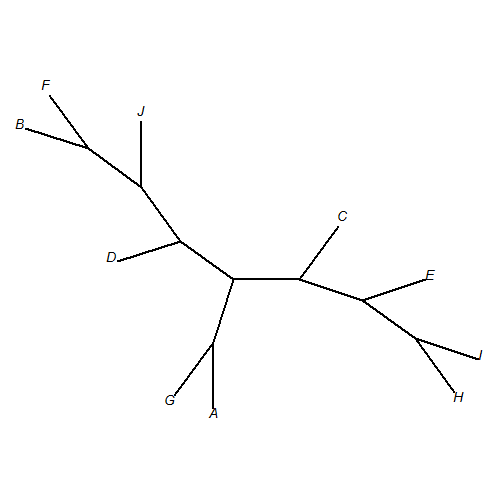``````trees<-list()
for(i in 1:4) trees[[i]]<-drop.tip(tree,sample(tree\$tip.label,5))
class(trees)<-"multiPhylo"
print(trees,details=TRUE)
``````
``````## 4 phylogenetic trees
## tree 1 : 5 tips
## tree 2 : 5 tips
## tree 3 : 5 tips
## tree 4 : 5 tips
``````
``````mrp.tree<-mrp.supertree(trees)
``````
``````##  "Best pscore so far: 10"
##  "Best pscore so far: 10"
##  "Best pscore so far: 10"
##  "Best pscore so far: 10"
##  "Best pscore so far: 10"
##  "Best pscore so far: 10"
##  "Best pscore so far: 10"
##  "Best pscore so far: 10"
##  "Best pscore so far: 10"
##  "Best pscore so far: 10"
``````
``````## The MRP supertree, optimized via pratchet(),
## has a parsimony score of 10 (minimum 10)
``````
``````plot(mrp.tree,type="unrooted",no.margin=TRUE,use.edge.length=FALSE,
edge.width=2,cex=1.2)
``````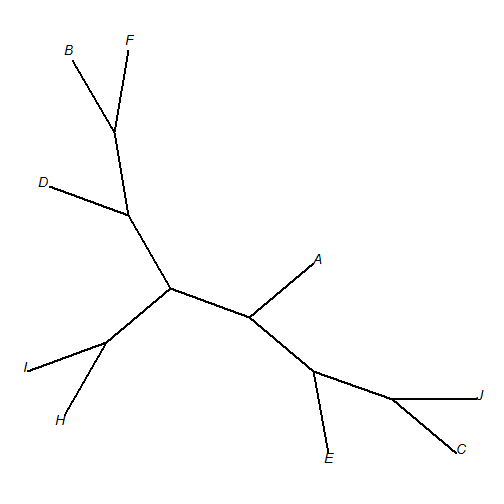``````library(phangorn)
RF.dist(drop.tip(tree,setdiff(tree\$tip.label,mrp.tree\$tip.label)),
mrp.tree)
``````
``````##  8
``````

And if we just want the matrix:

``````obj<-compute.mr(trees)
obj
``````
``````## 9 sequences with 10 character and 10 different site patterns.
## The states are 0 1
``````
``````obj<-compute.mr(trees,type="matrix")
obj
``````
``````##   [,1] [,2] [,3] [,4] [,5] [,6] [,7] [,8] [,9] [,10]
## A "1"  "0"  "1"  "1"  "?"  "?"  "?"  "1"  "1"  "0"
## H "1"  "1"  "?"  "?"  "1"  "1"  "1"  "?"  "?"  "?"
## I "1"  "1"  "?"  "?"  "1"  "1"  "1"  "?"  "?"  "?"
## F "0"  "0"  "0"  "0"  "?"  "?"  "?"  "?"  "?"  "?"
## B "0"  "0"  "0"  "0"  "?"  "?"  "?"  "0"  "0"  "0"
## D "?"  "?"  "1"  "0"  "?"  "?"  "?"  "1"  "0"  "0"
## C "?"  "?"  "1"  "1"  "1"  "0"  "0"  "1"  "1"  "1"
## E "?"  "?"  "?"  "?"  "1"  "1"  "0"  "1"  "1"  "1"
## J "?"  "?"  "?"  "?"  "0"  "0"  "0"  "?"  "?"  "?"
``````

That's really it!

This phytools development version can be installed from GitHub as follows:

``````library(devtools)
intall_github("liamrevell/phytools")
``````

## Wednesday, November 11, 2015

### Computing the relative frequencies of changes of different types along edges in a sample of stochastic map trees

I just added a function to phytools to compute the relative frequency of changes between states along edges in a sample of trees from stochastic mapping using `make.simmap`. This is similar to what I showed here, but with the added detail that the frequency of changes between all states are computed.

First, here is what the function looks like, as it is relatively simple:

``````edgeProbs <- function (trees)
{
if (!inherits(trees, "multiSimmap"))
stop("trees should be an object of class \"multiSimmap\".")
SS <- sapply(trees, getStates, "tips")
states <- sort(unique(as.vector(SS)))
m <- length(states)
TT <- sapply(states, function(x, y) sapply(y, paste, x, sep = "->"),
y = states)
nn <- c(TT[upper.tri(TT)], TT[lower.tri(TT)])
fn <- function(edge, trees, states) {
obj <- sapply(trees, function(x, e, s) if (names(x\$maps[[e]]) ==
s && names(x\$maps[[e]])[length(x\$maps[[e]])] ==
s)
TRUE
else FALSE, e = edge, s = states)
sum(obj)/length(obj)
}
edge.probs <- matrix(0, nrow(trees[]\$edge), m, dimnames = list(apply(trees[]\$edge,
1, paste, collapse = ","), nn))
k <- 1
for (i in 1:m) for (j in 1:m) {
if (i != j) {
edge.probs[, k] <- sapply(1:nrow(trees[]\$edge),
fn, trees = trees, states = states[c(i, j)])
k <- k + 1
}
}
edge.probs <- cbind(edge.probs, 1 - rowSums(edge.probs))
colnames(edge.probs)[ncol(edge.probs)] <- "no change"
edge.probs
}
``````

Now, let's see it in action. First, here are our trees:

``````trees
``````
``````## 100 phylogenetic trees with mapped discrete characters
``````

Let's plot them:

``````colors<-setNames(c("blue","red"),c("a","b"))
par(mfrow=c(10,10))
plot(trees,ftype="off",lwd=1,colors=colors)
``````Now let's use `edgeProbs` to compute the relative frequency of branches in which the mapped state changes from one value to another. Note that this only considers the states at flanking nodes, and does not count “multiple-hits” that occur along edges.

``````obj<-edgeProbs(trees)
obj
``````
``````##       a->b b->a no change
## 27,28 0.12 0.01      0.87
## 28,29 0.02 0.00      0.98
## 29,30 0.01 0.00      0.99
## 30,1  0.00 0.00      1.00
## 30,31 0.00 0.00      1.00
## 31,2  0.00 0.00      1.00
## 31,3  0.00 0.00      1.00
## 29,32 0.00 0.00      1.00
## 32,4  0.01 0.00      0.99
## 32,5  0.01 0.00      0.99
## 28,6  0.03 0.00      0.97
## 27,33 0.10 0.04      0.86
## 33,7  0.08 0.00      0.92
## 33,34 0.02 0.01      0.97
## 34,35 0.02 0.01      0.97
## 35,36 0.00 0.00      1.00
## 36,37 0.00 0.73      0.27
## 37,8  0.00 0.21      0.79
## 37,38 0.00 0.21      0.79
## 38,9  0.00 0.00      1.00
## 38,10 0.00 0.00      1.00
## 36,39 0.03 0.01      0.96
## 39,11 0.04 0.00      0.96
## 39,12 0.04 0.00      0.96
## 35,40 0.03 0.00      0.97
## 40,41 0.03 0.00      0.97
## 41,13 0.00 0.00      1.00
## 41,14 0.00 0.00      1.00
## 40,42 0.01 0.04      0.95
## 42,43 0.01 0.00      0.99
## 43,15 0.05 0.00      0.95
## 43,16 0.05 0.00      0.95
## 42,44 0.00 0.92      0.08
## 44,17 0.00 0.02      0.98
## 44,18 0.00 0.02      0.98
## 34,45 0.01 0.10      0.89
## 45,19 0.00 0.84      0.16
## 45,46 0.01 0.01      0.98
## 46,47 0.09 0.00      0.91
## 47,48 0.07 0.00      0.93
## 48,20 0.00 0.00      1.00
## 48,49 0.00 0.00      1.00
## 49,21 0.00 0.00      1.00
## 49,22 0.00 0.00      1.00
## 47,50 0.07 0.00      0.93
## 50,23 0.00 0.00      1.00
## 50,24 0.00 0.00      1.00
## 46,51 0.00 0.82      0.18
## 51,25 0.00 0.02      0.98
## 51,26 0.00 0.02      0.98
``````

Now we can overlay these probabilities on our original tree, or on randomly chosen stochastic map tree. Here I will only plot the rows in which the probability of change is non zero (we could also set a threshold of some value, like 0.05.)

``````plot(trees[[sample(1:length(trees),1)]],colors=colors)
ii<-which(obj[,"no change"]<1)
piecols<-setNames(c("red","blue","white"),colnames(obj))
piecols
``````
``````##      a->b      b->a no change
##     "red"    "blue"   "white"
``````
``````edgelabels(edge=ii,pie=obj[ii,],piecol=piecols,cex=0.7)
``````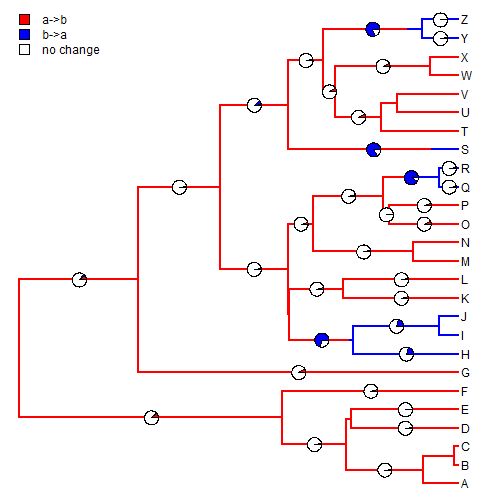Or, alternatively, with a threshold (and a different randomly chosen stochastic map):

``````plot(trees[[sample(1:length(trees),1)]],colors=colors)
ii<-which(obj[,"no change"]<0.95)
piecols<-setNames(c("red","blue","white"),colnames(obj))
piecols
``````
``````##      a->b      b->a no change
##     "red"    "blue"   "white"
``````
``````edgelabels(edge=ii,pie=obj[ii,],piecol=piecols,cex=0.9)
``````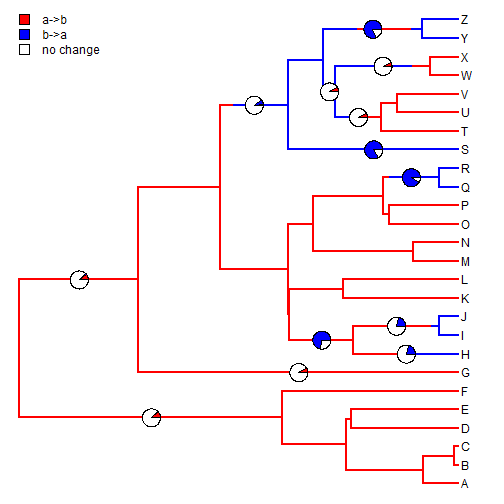To get a version of phytools with this feature you need to install from GitHub which can be done as follows:

``````library(devtools)
install_github("liamrevell/phytools")
``````

The data used in this demo were simulated as follows:

``````tree<-pbtree(n=26,tip.label=LETTERS,scale=1)
Q<-matrix(c(-1,1,1,-1),2,2,dimnames=list(letters[1:2],letters[1:2]))
x<-sim.history(tree,Q)\$states
trees<-make.simmap(tree,x,nsim=100)
``````

That's all for now!

## Saturday, November 7, 2015

### Computing the frequency of changes along edges of a set of stochastic map trees

In response to a recent R-sig-phylo thread I thought it might be useful to post code demonstrating how to compute the relative frequency of changes of particular types along edges in a posterior sample from the method of stochastic mapping.

``````## load libraries
library(phytools)
## here is our data
tree
``````
``````##
## Phylogenetic tree with 26 tips and 25 internal nodes.
##
## Tip labels:
##  A, B, C, D, E, F, ...
##
## Rooted; includes branch lengths.
``````
``````x
``````
``````##   A   B   C   D   E   F   G   H   I   J   K   L   M   N   O   P   Q   R
## "a" "a" "a" "b" "b" "b" "b" "b" "b" "a" "a" "a" "b" "a" "a" "a" "a" "a"
##   S   T   U   V   W   X   Y   Z
## "a" "a" "a" "b" "b" "b" "b" "b"
``````
``````## obtain stochastic maps:
trees<-make.simmap(tree,x,model="ER",nsim=100)
``````
``````## make.simmap is sampling character histories conditioned on the transition matrix
##
## Q =
##           a         b
## a -2.818303  1.409151
## b  1.409151 -2.818303
## (estimated using likelihood);
## and (mean) root node prior probabilities
## pi =
##   a   b
## 0.5 0.5
``````
``````## Done.
``````

Now let's do our analysis. The following function computes for a given edge (by row in `tree\$edge`, but we could easily modify to be by node number) the relative frequency of trees in which the state at the start of the edge is `"a"` and the state at the end is `"b"`.

``````## this function computes for a given edge
foo<-function(edge,trees,states){
obj<-sapply(trees,function(x,e,s)
if(names(x\$maps[[e]])==s&&names(x\$maps[[e]])[length(x\$maps[[e]])]==s) TRUE
else FALSE,e=edge,s=states)
sum(obj)/length(obj)
}
``````

We can use it by iterating over edges:

``````edge.probs<-sapply(1:nrow(tree\$edge),foo,trees=trees,states=c("a","b"))
edge.probs
``````
``````##   0.09 0.21 0.00 0.00 0.00 0.00 0.00 0.53 0.00 0.27 0.43 0.02 0.01 0.01
##  0.00 0.00 0.00 0.00 0.01 0.00 0.01 0.01 0.00 0.00 0.02 0.00 0.97 0.00
##  0.00 0.00 0.00 0.00 0.00 0.00 0.00 0.13 0.05 0.00 0.00 0.00 0.00 0.00
##  0.41 0.00 0.00 0.40 0.03 0.03 0.00 0.00
``````

Finally, let's visualize the results on a 'representative' stochastic map:

``````plot(trees[],colors=setNames(c("blue","red"),c("a","b")),
ylim=c(-2,Ntip(tree)))
par(fg="transparent") ## just for clarity
threshold<-0 ## threshold frequency above which we want to plot it
edgelabels(edge=which(edge.probs>threshold),
pie=edge.probs[which(edge.probs>threshold)],
piecol=c("red","black"),cex=0.8)
par(fg="black")
add.simmap.legend(colors=setNames(c("red","black"),c("freq. change from a to b",
"freq. no change or change from b to a")),prompt=FALSE,x=0,y=0)
``````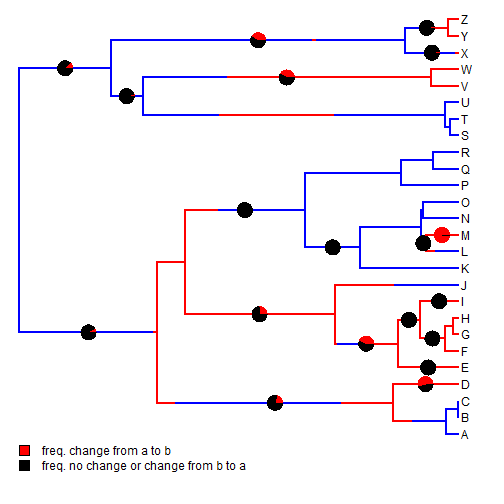Or we can change the threshold to `0.05`:

``````plot(trees[],colors=setNames(c("blue","red"),c("a","b")),
ylim=c(-2,Ntip(tree)))
par(fg="transparent") ## just for clarity
threshold<-0.05 ## threshold frequency above which we want to plot it
edgelabels(edge=which(edge.probs>threshold),
pie=edge.probs[which(edge.probs>threshold)],
piecol=c("red","black"),cex=0.8)
par(fg="black")
add.simmap.legend(colors=setNames(c("red","black"),c("freq. change from a to b",
"freq. no change or change from b to a")),prompt=FALSE,x=0,y=0)
``````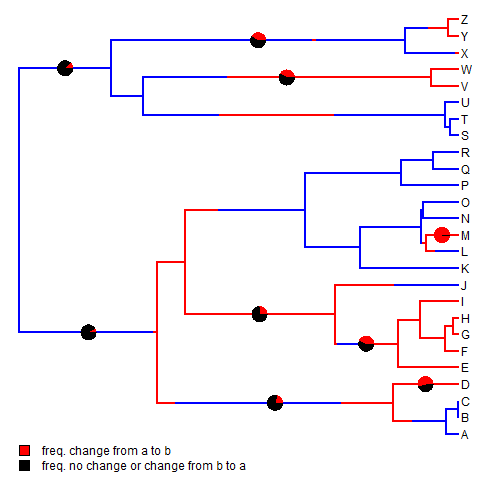``````tree<-pbtree(n=26,tip.label=LETTERS,scale=1)